Posted YEN_csdn

# 概率

0 <= P <= 1

• 根据个人置信
• 根据历史数据
• 根据模拟数据

## 条件概率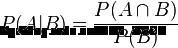# Logistic Regression (逻辑回归)

## 例子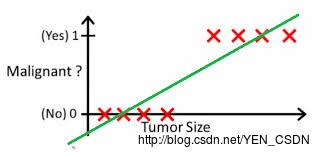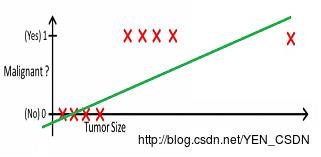## 基本模型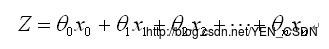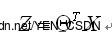（*）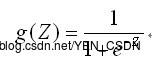（**）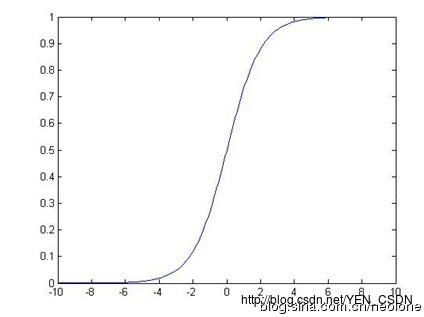用这个函数来模拟0-1之间的变化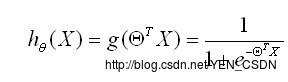（y==1）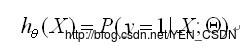(X为自变量，Θ为待求参数，所以此表达式的意思为：给定X，Θ的情况下y=1的概率为多少)
（y==0）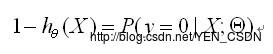（所以此表达式的意思为：给定X，Θ的情况下y=1的概率为多少）

## Cost函数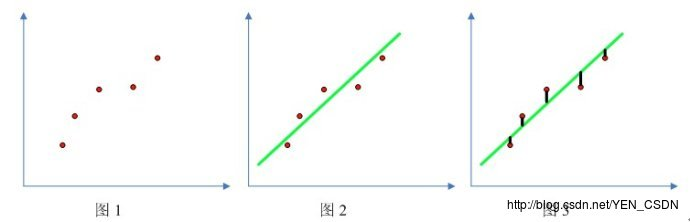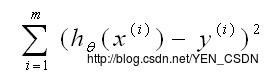• m:m个实例
• y(i):第i个实例的真实值为多少
• x(i):每个实例自变量是多少
• h(x(i)):每个实例自变量套用h(z)这个方程的函数值，即预测值（y_hat）

### 在线性方程中Cost函数：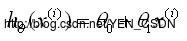### 在回归方程中Cost函数：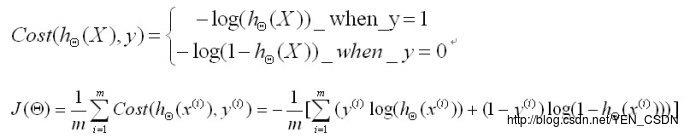（要找最小，所以加了-号；取对数方便加减、求导；把y=1时和y=0时的方程合并为J(Θ) ）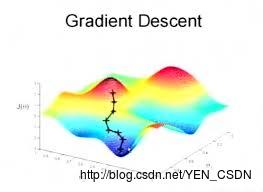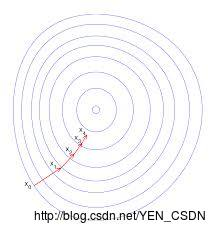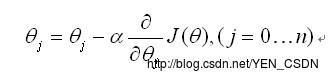J(Θ)对Θj求偏导
alpha：学习率，就是每一步的步长

### 更新法则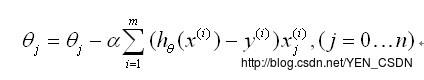• alpha:学习率
• i：不同的实例
• 同时对所有Θ进行更新，重复更新直至收敛（小于设定的阈值）

``````#coding=utf-8

# @Author: yangenneng
# @Time: 2018-01-18 15:30
# @Abstract：非线性回归-逻辑回归算法

import numpy as np
import random

'''
# function:产生一些数据，用来做拟合
# numPoints：实例个数
# bias：随机生成y时的偏好
# variance：一组数据的方差
'''
def genData(numPoints,bias,variance):
# 生成numPoints行2列的零矩阵  shape形状
x = np.zeros(shape=(numPoints, 2))
y = np.zeros(shape=(numPoints))
# 循环numPoints次，及i=0 到 numPoints-1
for i in range(0,numPoints):
x[i] = 1
x[i] = i
# random.uniform(0,1)  从0-1之间随机产生一些数
y[i] = (i+bias)+random.uniform(0, 1) * variance
return x, y

'''
# 梯度下降算法
# x：自变量矩阵，每行表示一个实例
# y：实例的真实值
# theta：待求的参数
# alpha：学习率
# m：总共m个实例
# numIterations：重复更新的次数（重复更新直至收敛（小于设定的阈值））
'''
# 矩阵转置
xTran = np.transpose(x)
# 循环次数
for i in range(0,numIterations):
# 公式中的Z
hypothesis = np.dot(x,theta)
# loss:预测值-实际值
loss = hypothesis-y
# cost就是公式中J(Θ)，这里定义的是一个简单的函数
cost = np.sum(loss ** 2) / (2 * m)
# 每次更新的更新量，即更新法则
# Θ
print ("Iteration %d | cost :%f" % (i, cost))
return theta

# 参数数据
x,y = genData(100, 25, 10)
print "x:", x
print "y:", y

# 查看产生数据的行列
m,n = np.shape(x)
n_y = np.shape(y)
print("m:"+str(m)+" n:"+str(n)+" n_y:"+str(n_y))

# 求Θ
numIterations = 100000
alpha = 0.0005
theta = np.ones(n)
theta= gradientDescent(x, y, theta, alpha, m, numIterations)
print(theta)

``````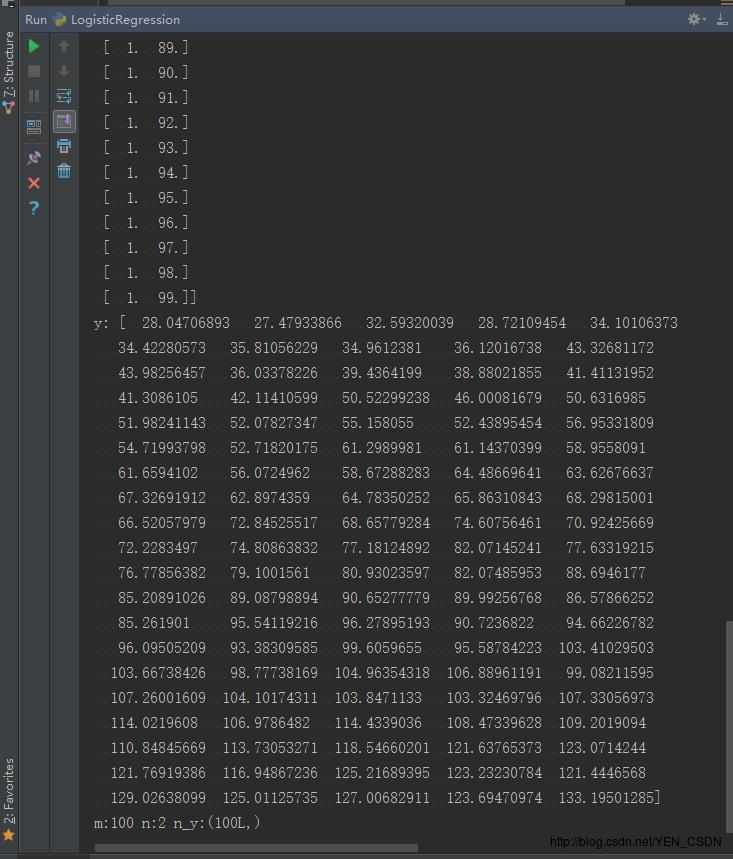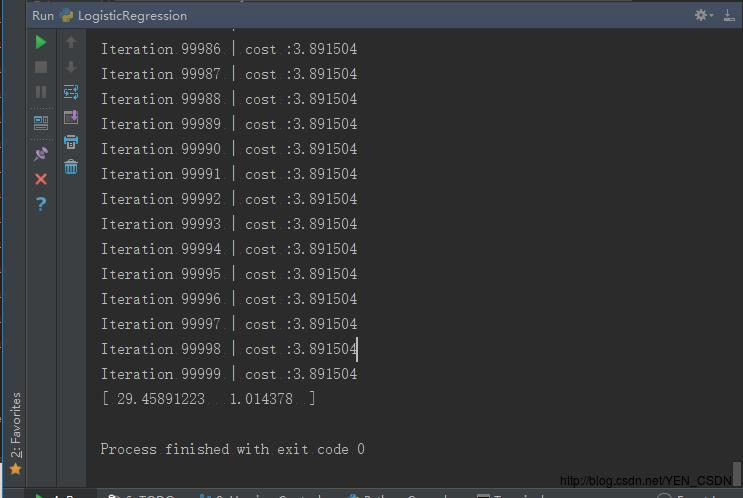[ 29.45891223 1.014378 ]表示Θ1、Θ2（因为X是二维的）